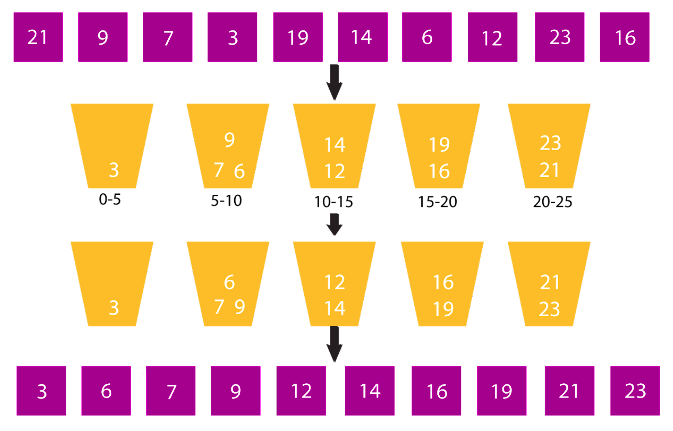# Bucket Sort

### Introduction

To grasp the concept of bucket sort, it is essential to first understand the technique and the series of operations required to make the array sorted using counting sort.

In this blog, we have also used an example and its code snippet to understand the concept better.

## Working of Bucket sort

After dividing the elements, each bucket can use any of the sorting algorithms or recursively apply the same bucket algorithm to sort elements within the bucket.

The sorted buckets are finally combined and made into a sorted array.### Bucket Sort Algorithm

• The array ‘arr[]’ with ‘N’ size is considered. For sorting the array, ‘N’ number of buckets are created.
• Elements from the array are then put into buckets. The buckets are then sorted individually.
• After sorting the elements inside the bucket, the buckets are combined again to form the sorted array.
• The function is then called for the input array.

### Code snippet:

#### Time Complexity

The time complexity depends mainly on the range of elements and the size of the bucket list. Elements not having a wide range would mean a lot of elements being stored in the same bucket and increasing the complexity. For a total of ‘K’ buckets, the outer loop will take O(K) time and the inner loop will take O(N) time. So the time complexity of bucket sort is O(N * K) which will be O(N * N) in the worst case.

#### Space Complexity

The space complexity is O(N + K). As ‘N’ buckets of size ‘K’ are created into which total ‘N’ elements will be placed which will result in the space complexity of O(N + K) or O(N).

When should bucket sort be used?

Bucket sort can be best put to use when data is distributed over a range uniformly, such as in a large array of integers.

Is bubble sort faster than bucket sort?

Dividing data into small buckets capable of being stored individually means lesser comparisons are needed. Therefore a significant advantage of bucket sort is being faster than bubble sort.

Can bucket sort be used to sort strings?

Bucket sort cannot be used to sort arbitrary strings, but can efficiently sort a set of uniformly distributed floating-point numbers in an array.

What is the difference between bucket sort and counting sort?

Counting sort stores single elements in a bucket, i.e., the count of items. Bucket sort, on the other hand, requires dynamic arrays, linked lists or pre-allocated memory to hold collections of elements in every bucket.

What is the disadvantage of bucket sort?

Bucket sort cannot be used for all data types because it requires the ideal bucketing scheme. Moreover, it is inefficient in cases with tightly clustered values because of its sensitivity towards the distribution of input.

### Key takeaways

In this article, we learned how the bucket sort algorithm works and how it is implemented, along with an example.

The bucket sort technique is very efficient for cases where input values where the range of elements is wide. You can go to CodeStudio and try solving problems. Share this blog with your friends if you found it helpful!

Until then, All the best for your future endeavours, and Keep Coding.

By Reet Maggo